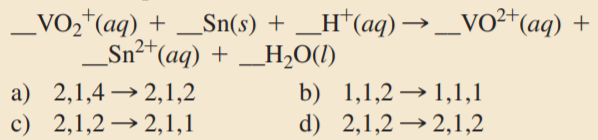×
Get Full Access to Chemistry: A Molecular Approach - 3 Edition - Chapter 18 - Problem 1saq
Get Full Access to Chemistry: A Molecular Approach - 3 Edition - Chapter 18 - Problem 1saq

×

# Balance the redox reaction equation (occurring in acidicISBN: 9780321809247 1

## Solution for problem 1SAQ Chapter 18

Chemistry: A Molecular Approach | 3rd Edition

• Textbook Solutions
• 2901 Step-by-step solutions solved by professors and subject experts
• Get 24/7 help from StudySoup virtual teaching assistantsChemistry: A Molecular Approach | 3rd Edition

4 5 1 371 Reviews
19
5
Problem 1SAQ

Balance the redox reaction equation (occurring in acidic solution) and choose the correct coefficients for each reactant and product.Step-by-Step Solution:
Step 1 of 3

Step 2 of 3

Step 3 of 3

##### ISBN: 9780321809247

The full step-by-step solution to problem: 1SAQ from chapter: 18 was answered by , our top Chemistry solution expert on 02/22/17, 04:35PM. This full solution covers the following key subjects: acidic, balance, choose, coefficients, correct. This expansive textbook survival guide covers 82 chapters, and 9454 solutions. The answer to “?Balance the redox reaction equation (occurring in acidic solution) and choose the correct coefficients for each reactant and product.” is broken down into a number of easy to follow steps, and 19 words. Chemistry: A Molecular Approach was written by and is associated to the ISBN: 9780321809247. This textbook survival guide was created for the textbook: Chemistry: A Molecular Approach, edition: 3. Since the solution to 1SAQ from 18 chapter was answered, more than 308 students have viewed the full step-by-step answer.

Unlock Textbook Solution